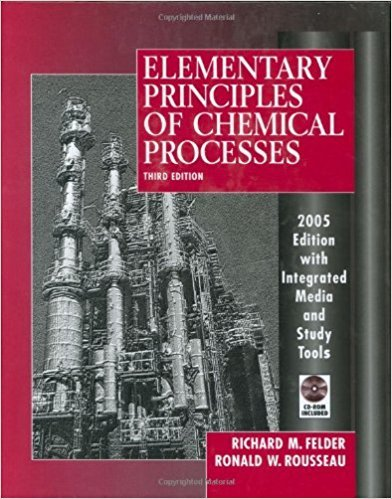×
Get Full Access to Elementary Principles Of Chemical Processes - 3 Edition - Chapter 8 - Problem 8.90
Get Full Access to Elementary Principles Of Chemical Processes - 3 Edition - Chapter 8 - Problem 8.90

×

# Two hundred kilograms per hour of an aqueous solutionISBN: 9780471687573 143

## Solution for problem 8.90 Chapter 8

Elementary Principles of Chemical Processes | 3rd Edition

• Textbook Solutions
• 2901 Step-by-step solutions solved by professors and subject experts
• Get 24/7 help from StudySoup virtual teaching assistantsElementary Principles of Chemical Processes | 3rd Edition

4 5 1 308 Reviews
14
3
Problem 8.90

Two hundred kilograms per hour of an aqueous solution containing 20.0 mole% sodium acetate (NaC2H30 Z) enters an evaporative crystallizer at 60C. When the solution is exposed to the low pressure in the evaporator, 16.9% of the water evaporates, concentrating the remaining solution and causing crystals of sodium acetate trihydrate (NaC2H3 0 23 H20) to form. The product is an equilibrium mixture of crystals and a saturated aqueous solution containing 15.4 mole % NaC2H3 0 2. The effluents (crystals, solution, and water vapor) are all at 50C. (a) Calculate the feed rate to the crystallizer in kmol/h. (b) Calculate the production rate (kglh) of trihydrate crystals and the mass flow rate (kg/h) of the liquid solution in which the crystals are suspended. (c) Estimate the rate (kJ/h) at which heat must be transferred to or from the crystallizer (state which), using the following physical property data: (Cp)all solutions = 3.5 kJ/(kg'C) (Cp)crystals = 1.2 kJ/(kg'C) (Cp)HzO(Y) = 32.4 kJ/(kmol'C) (MfY)HzO = 4.39 X 104 kJ/kmol Heat of solution of anhydrous sodium acetate: Mfs(25C) = -1.71 X 104 kJ/kmol NaC2H30 2 Heat of hydration: NaC2H30 2 (S) + 3 H2 0(I) - NaC2H3 0 23 H2 0(s) tlH(25C) = -3.66 X 104 kJ/kmol NaC2H30 2

Step-by-Step Solution:
Step 1 of 3

9/19 *​Notes from last ch​apter: When naming Binary Ions, Zinc and Silver are the only transition metals that do not need Roman Numerals, because Zinc is always Zn​ and Silver is always Ag​ . Also, Aluminum is ​ always Al​ . +​ *We are devoting so much time to this chapter because ​naming is a good portion of the first test​. CHAPTER 3­ Compositions of Substances and Solutions Ratios and Stoichiometry 1) Na​ P3​ : 4​ams are no use for comparison in elements 2) Moles and the number of atoms are the key to relating products to reactants, we need numbers for things The Mole 1) How much is a mole a) A mole of pennies would be a stack 300 meters tall. 2) 1.00 mol of atoms is ​6.022 × 10​

Step 2 of 3

Step 3 of 3

##### ISBN: 9780471687573

Unlock Textbook Solution